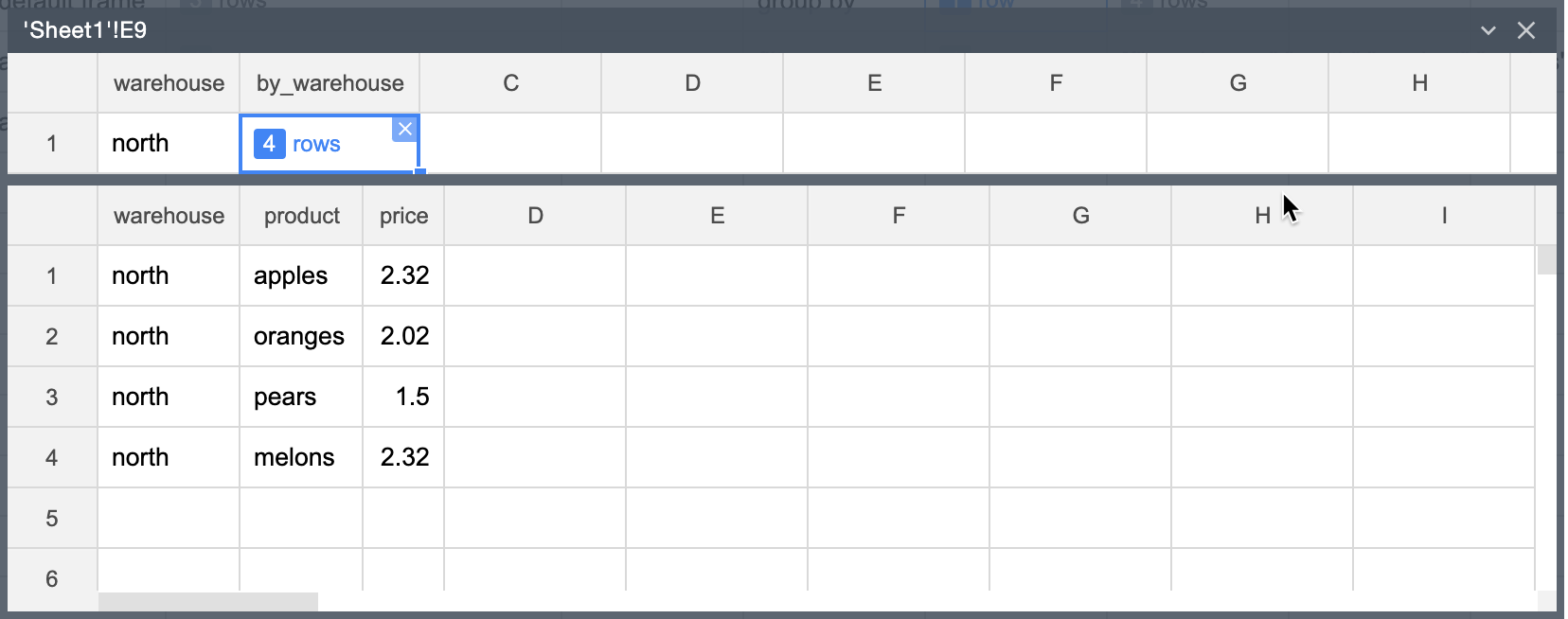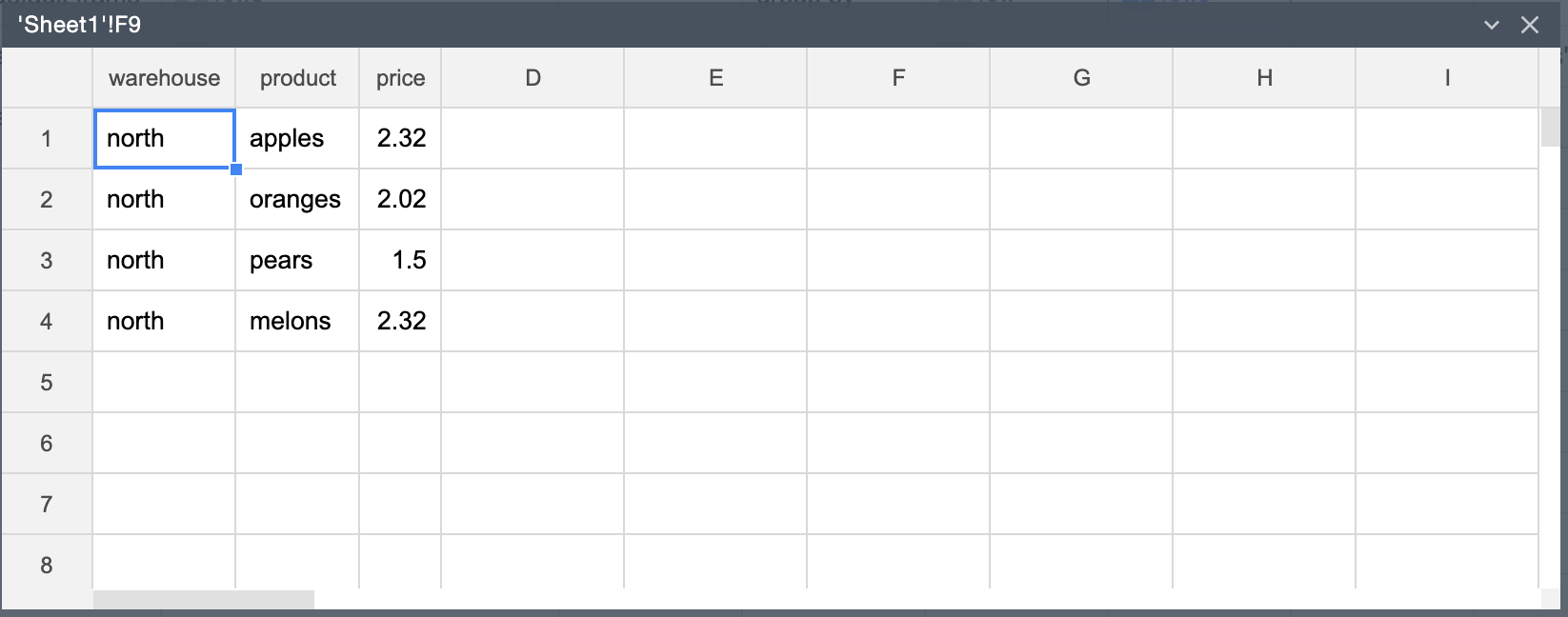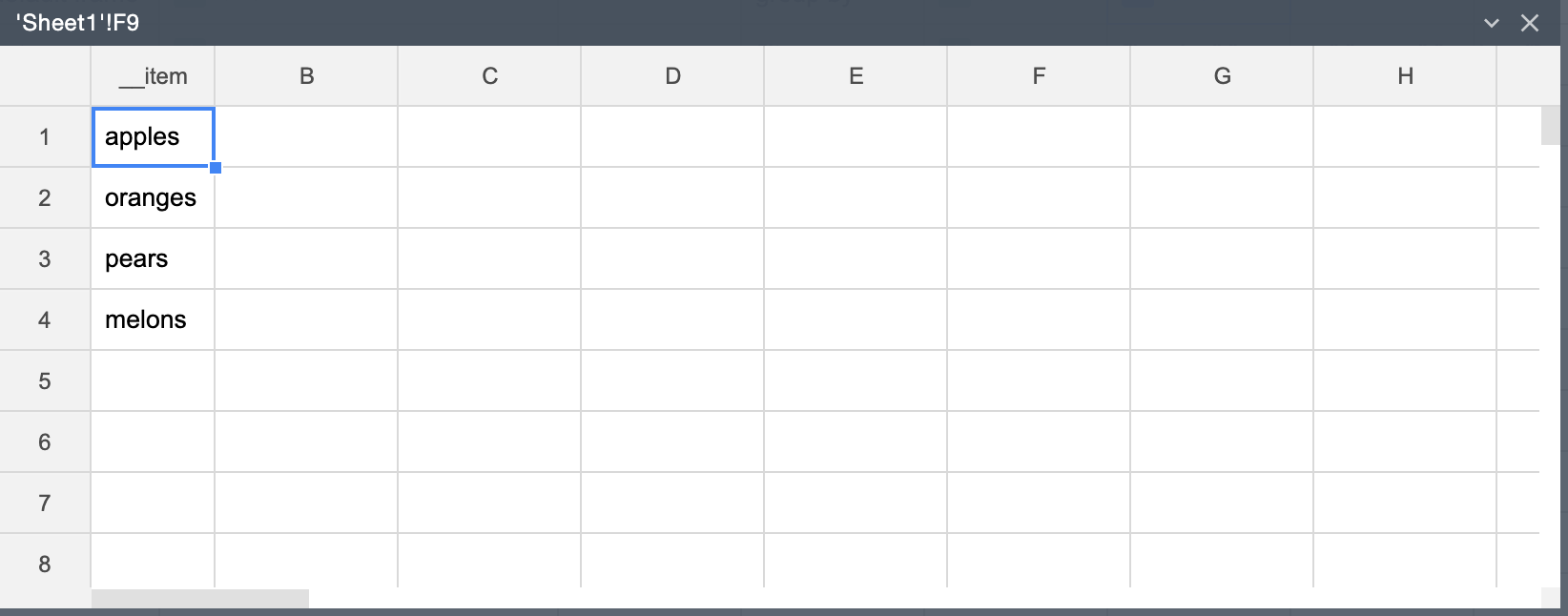Learn

# Retrieve rows

By using the GRAB() function you can retrieve values from a frame regardless of how deep the values are nested in the frame.

To specify values to retrieve, use a JSONPath-like expression in the format \$..{column}..{nested_column}.

Let's take as an example the below nested frame created using the GROUP_BY() function.• To retrieve the "by_warehouse" values from it, use the following formula:
=GRAB(E9,"\$..by_warehouse")Where E9 is the address of the cell containing the frame.

The resulting frame is shown in the figure below.• To retrieve the "product" values, use the following formula:
=GRAB(E9,"\$..product")Where E9 is the address of the cell containing the frame.

The resulting frame is shown in the figure below.• To retrieve the third row from the "product" values, use the following formula:
=GRAB(E9,"\$...product")Where E9 is the address of the cell containing the frame.

# Try it now

Sorry, your browser is not supported. MINTDATA™ works best on Google Chrome 50.0+ and Firefox 44.0+.
Contents